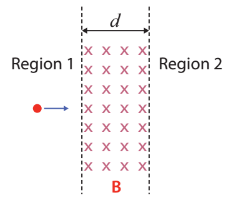# Problem: Two regions in space, Region 1 and Region 2, are separated by a gap of thickness  d filled with a magnetic field of B pointing into the page, as shown in the figure.  If d = 1 cm and B = 0.1 T, what is the minimum kinetic energy required for an electron to penetrate from Region 1 into Region 2? (1) 1.4 × 10−14 J (2) 2.8 × 10−14 J (3) 2.1 × 10−14 J (4) 3.5 × 10−14 J (5) 4.2 × 10−14 J

###### FREE Expert Solution
89% (166 ratings)
###### Problem Details

Two regions in space, Region 1 and Region 2, are separated by a gap of thickness  d filled with a magnetic field of B pointing into the page, as shown in the figure.  If d = 1 cm and B = 0.1 T, what is the minimum kinetic energy required for an electron to penetrate from Region 1 into Region 2?

(1) 1.4 × 10−14 J

(2) 2.8 × 10−14 J

(3) 2.1 × 10−14 J

(4) 3.5 × 10−14 J

(5) 4.2 × 10−14 J